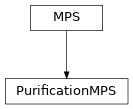# purification_mps¶

ClassesPurificationMPS(sites, Bs, SVs[, bc, form, norm]) An MPS representing a finite-temperature ensemble using purification.

Module description

This module contains an MPS class representing an density matrix by purification.

Usually, an MPS represents a pure state, i.e. the density matrix is $$\rho = |\psi><\psi|$$, describing observables as $$<O> = Tr(O|\psi><\psi|) = <\psi|O|\psi>$$. Clearly, if $$|\psi>$$ is the ground state of a Hamiltonian, this is the density matrix at T=0.

At finite temperatures $$T > 0$$, we want to describe a non-pure density matrix $$\rho = \exp(-H/T)$$. This can be accieved by the so-called purification: in addition to the physical space P, we introduce a second ‘auxiliar’ space Q and define the density matrix of the physical system as $$\rho = Tr_Q(|\phi><\phi|)$$, where $$|\phi>$$ is a pure state in the combined phyisical and auxiliar system.

For $$T=\infty$$, the density matrix $$\rho_\infty$$ is the identity matrix. In other words, expectation values are sums over all possible states $$<O> = Tr_P(\rho_\infty O) = Tr_P(O)$$. Saying that each : on top is to be connected with the corresponding : on the bottom, the trace is simply a contraction:

|         :   :   :   :   :   :
|         |   |   |   |   |   |
|         |-------------------|
|         |        O          |
|         |-------------------|
|         |   |   |   |   |   |
|         :   :   :   :   :   :


Clearly, we get the same result, if we insert an identity operator, written as MPO, on the top and bottom:

|         :   :   :   :   :   :
|         |   |   |   |   |   |
|         B---B---B---B---B---B
|         |   |   |   |   |   |
|         |-------------------|
|         |        O          |
|         |-------------------|
|         |   |   |   |   |   |
|         B*--B*--B*--B*--B*--B*
|         |   |   |   |   |   |
|         :   :   :   :   :   :


We use the following label convention:

|         q
|         ^
|         |
|  vL ->- B ->- vR
|         |
|         ^
|         p


You can view the MPO as an MPS by combining the p and q leg and defining every physical operator to act trivial on the q leg. In expecation values, you would then sum over over the q legs, which is exactly what we need. In other words, the choice $$B = \delta_{p,q}$$ with trivial (length-1) virtual bonds yields infinite temperature expectation values for operators action only on the p legs!

Now, you go a step further and also apply imaginary time evolution (acting only on p legs) to the initial infinite temperature state. For example, the normalized state $$|\psi> \propto \exp(-\beta/2 H)|\phi>$$ yields expecation values

$<O> = Tr(\exp(-\beta H) O) / Tr(\exp(-\beta H)) \propto <\phi|\exp(-\beta/2 H) O \exp(-\beta/2 H)|\phi>.$

An additional real-time evolution allows to calculate time correlation functions:

$<A(t)B(0)> \propto <\phi|\exp(-\beta H/2) \exp(+i H t) A \exp(-i H t) B \exp(-\beta H/2) |\phi>$

Time evolution algorithms (TEBD and MPO application) are adjusted in the module purification.

See also [karrasch2013] for additional tricks! On of their crucial observations is, that one can apply arbitrary unitaries on the auxiliar space (i.e. the q) without changing the result. This can actually be used to reduce the necessary virtual bond dimensions: From the definition, it is easy to see that if we apply $$exp(-i H t)$$ to the p legs of $$|\phi>$$, and $$\exp(+iHt)$$ to the q legs, they just cancel out! (They commute with $$\exp(-\beta H/2)$$…) If the state is modified (e.g. by applying A or B to calculate correlation functions), this is not true any more. However, we still can find unitaries, which are ‘optimal’ in the sense of reducing the entanglement of the MPS/MPO to the minimal value. For a discussion of Disentanglers (implemented in disentanglers), see [hauschild2018].

Note

The classes MPSEnvironment and TransferMatrix should also work for the PurificationMPS defined here. For example, you can use expectation_value() for the expectation value of operators between different PurificationMPS. However, this makes only sense if the same disentangler was applied to the bra and ket PurificationMPS.

Note

The literature (e.g. section 7.2 of [schollwoeck2011] or [karrasch2013]) suggests to use a singlet as a maximally entangled state. Here, we use instead the identity $$\delta_{p,q}$$, since it is easier to generalize for p running over more than two indices, and allows a simple use of charge conservation with the above qconj convention. Moreover, we don’t split the physical and auxiliar space into separate sites, which makes TEBD as costly as $$O(d^6 \chi^3)$$.

Todo

One can also look at the canonical ensembles by defining the conserved quantities differently, see [barthel2016] for details. Idea: usual charges on p, trivial charges on q; fix total charge to desired value. I think it should suffice to implement another from_infiniteT.